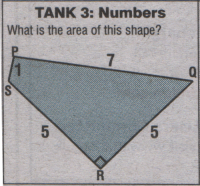# Metro PuzzleI try to stimulate my tired brain every morning attempting the Metro Puzzle on the way in to work, today seemed to be a simple maths problem, and after attempting to guess the answer, I checked to see if I was correct.
Now not to spoil it for you, I’ve put my answer and their answer in the comments sections.

## 4 thoughts on “Metro Puzzle”

1.Ryan says:

Here’s what I did:
RSQ is a right angled triangle, so using Pythagoras the length SQ is ƒ50.
Now a quick bit of maths shows that 7² + 1² = 50, which means that SPQ must also be a right angled triangle.
All thats left to do is calculate the area.
Area of RSQ = ½.5.5 = 12.5 units²
Area of SPQ = ½.1.7 = 3.5 units²
Total Area = 16 units²

However metro, who use the same calculations to deduce that we have two right angle triangles, say
“Pythagroras’ Theorem twice gives area = 50 units²”

For annonation ƒ represents the square root of

2.Will says:

I did it your way to get 16 units². I don’t know what they’re wittering about. 50units² would be a shape 4 times the size of RSQ.

3.Ryan says:

Metro today corrected themselves over this error 🙂

4.Anonymous says:

wazzup